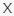# Can you pass this maths quiz? Answers

Q 1. Let’s start with an easy one. Can you find the correct solution?

Choices:

4
8
12
16

4

Choices:

3
9
12
15

9

Q 3. Let’s try some subtraction!

Choices:

50
100
150
200

50

Q 4. Can you solve this equation?

Choices:

1
13
19
16

16

Q 5. Double 15 and what do you get?

Choices:

20
30
40
50

30

Q 6. Can you find the correct solution?

Choices:

1
2
11
12

1

Choices:

23
27
29
25

25

Q 8. Can you solve this multiplication?

Choices:

72
88
80
64

64

Q 9. Do you know the answer?

Choices:

42
30
16
36

36

Q 10. What’s the correct solution?

Choices:

43
23
333
33